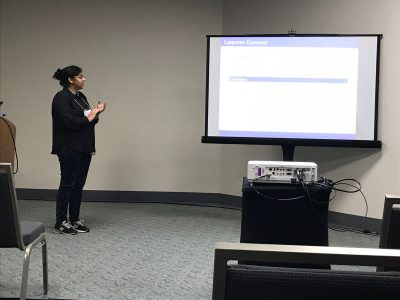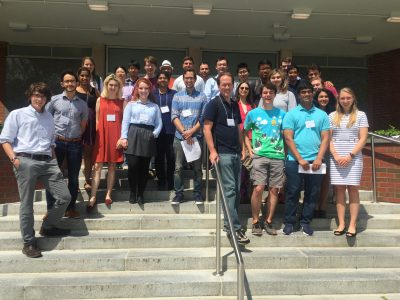# REU Seminars 2021

### July 23rd: Masha Gordina, University of Connecticut

Title: Emmy Noether, Symmetry and the Calculus of Variations

Title: TBA

Abstract:

### July 9th: Sasha Teplyaev, University of Connecticut

Title: Spectral Dimension of the Universe

Abstract:
We know that our usual space-time is four dimensional, but is it the same on a quantum scale? There is a recent physics theory that there is a so called dynamical dimensional reduction in quantum gravity. This talk will describe the basic mechanism for this dimension reduction, and a surprising connection to the talk by Chris Hayes.

### July 2nd: Patricia Alonso-Ruiz, Texas A&M

Title: Fractals are also in the market

Abstract: How does a financial investor decide which trading strategy to apply? Analyzing and classifying financial data is crucial to make a decision. One general classification involves two types of price behaviors: trending or mean-reverting. In the former, increasing (or decreasing) returns will likely lead to further increasing (or decreasing) returns, while in the latter price increases will likely lead to price decreases (or vice versa).
In this talk we will discuss a way to mathematically describe these regimes and discover that fractals play a fundamental role in that description.

### June 25th: Chris Hayes, University of Connecticut

Title: Fractals and Dirichlet forms

Abstract: We will explore some simple examples of fractals, discuss dimensions of self-similar sets and then look at the basic ingredient in doing analysis (calculus) on some fractals: the construction of a self-similar Dirichlet form.

### June 18th: Emily Gunawan, University of Oklahoma

Title: Box-ball systems and Tableaux

Abstract: Box-ball systems give a combinatorial model of a soliton, or solitary wave. We discuss some results obtained by past REU students in this area.

### June 11th: Oleksii Mostovyi, University of Connecticut

Title: Introduction to financial mathematics

Abstract: We introduce some foundational ideas of financial mathematics, including the first and second fundamental theorems of asset pricing.

### June 4th: Luke Rogers, University of Connecticut

Title: Measurement

Abstract: Since its earliest days, mathematics has been used to measure things. Usually so that someone could tax them! But measuring even simple things like the length of a curve in the plane can present many challenges. We will walk through some of the difficulties one might encounter and learn a little about the mathematics that arises from them.

# 2020 Math REU Conference

Math REU groups from Amherst College, UConn, UMass, Williams College and Yale University are getting together online on Thursday July 30th for a conference to share their summer research work.  The conference website is at

2020 Math REU Conference

# 2020 Math REU Conference

Math REU groups from Amherst College, UConn, UMass, Williams College and Yale University are getting together online on Thursday July 30th for a conference to share their summer research work.  The conference website is at

2020 Math REU Conference

# REU Seminars 2020

### July 17th: Luke Rogers, UConn

Can I measure it with mathematics?

### July 10th: Emily Meehan, Gallaudet University

Title: Baxter posets.

### July 3rd: Daniel Labardini-Fragoso, UNAM

Title: Introduction to Hyperbolic Geometry

### June 26th: Masha Gordina, University of Connecticut

Title: Isoperimteric inequalities

Abstract: The classical isoperimetric problem asks: among all figures with a given perimeter, which one encloses the greatest area? There are many different proofs of the isoperimetric inequality and interesting stories about the authors of these proofs. We will review different tools that can be used to prove such an inequality (plane geometry, calculus of variations and maybe even Fourier series), and if time permits we’ll describe how this type of problem is still an active area of research in analysis and geometry.

### June 19th: Ivan Contreras, Amherst College

Dynamics and Topology on Graphs.

### June 12th: Nadia Lafrenière, Dartmouth University

Derangements and their use in research (Algebraic Combinatorics)

### June 5th: Greg Muller, University of Oklahoma

Title: Counting paths with linear algebra

Abstract: Counting paths in a graph is an elementary but important problem with many applications. Something remarkable happens if you assemble these counts into a matrix: the determinants of submatrices count certain collections of paths. This simple observation has a simple proof, but wide-ranging applications. Time permitting, I will review some applications to Pascal’s triangle, matrix factorizations, total positivity, and electrical networks.

# REU Sigma Seminar 2019

Seminars convene every Thursday during the REU program at 12:30 pm in MONT 214.

August 1st at 10:30 am: Keith Conrad, The Biggest Known Prime Number.

Abstract: Since there are infinitely many prime numbers, there is no biggest prime. But there is always a biggest known prime, currently over 24 million digits. It belongs to a family of primes called Mersenne primes. The talk will discuss the history of Mersenne primes and how their primality is checked.

July 25: Gianmarco Molino, Heat Kernels and Index Theory.

Abstract: TBA.

July 18: Matthew Badge, Curves, and Parameterizations.

Abstract: I will introduce some ideas in metric geometry. A curve is the image of a continuous map from [0,1] into a metric space. How do we measure the length of a curve? How do we tell whether or not a set of points is a curve of finite length, and if it is, how do we build a map whose image is the curve? If time permits, I will also describe analogues of these questions for higher-dimensional curves, which are open for research.

July 11: Behrang Forghani, Harmonic Functions, and Trees.

Abstract: In this talk, I will introduce the notion of bounded harmonic functions and discuss some of their properties for Markov chains.
D. Blackwell classified the space of bounded harmonic functions for random walks on Z. I will present his elegant proof and other results related to regular trees.

July 4: No Seminar.

June 27: Arthur Parzygnat, Computing the square root of a positive matrix.

Abstract: Given any function f on some domain and a diagonalizable nxn matrix A whose eigenvalues are in the domain of f, we will prove there exists a polynomial p such that p(A)=Pf(D)P^{-1}, where P is a matrix of eigenvectors of A and D is the corresponding matrix of eigenvalues. This may sound strange when you look at examples. For instance, given a (positive) matrix A, we will find a polynomial p such that p(A)=sqrt(A), the square-root of A. Along the way of proving this theorem, we will learn several useful techniques and results. For example, we will prove that if B is a matrix that commutes with A, i.e. AB=BA, then f(A)B=Bf(A) for any function f (satisfying the above assumptions).

June 20: Reed Solomon, Graduate School Panel.

Abstract: Want to learn about graduate school in mathematics and the application process. Ask Professor Reed Solomon (Director of Graduate Studies) and Ph.D. students Waseet Kazmi, Gianmarco Molino, Sean Eustace, and Lisa Naples!

June 13: Christopher Hayes, Introduction to self-similar structures.

Abstract: One category of a fractal is the self-similar set. Famous examples include the Sierpinski Carpet and Koch Curve and analysis of these types of fractals is currently an active field of research. In this talk we will go over a variety of examples, discuss some construction methods, definitions, commonly used properties such as the open set condition and having a finite post-critical set, and end with comments on how analysis can be done on these sets, in particular, the construction of a Dirichlet form on a post-critically finite set.

# UCONN Math REU at REU Conference at UMass Amherst 2019

Our REU participants will be giving talks on July 23rd, 2019 at the REU Conference at UMass Amherst 2019.

Fractals projects:

• Resistance scaling on the Octacarpet  (Claire Canner, William Huang, Michael Orwin)
• Decimation structure of the spectra of self-similar groups (Brett Hungar, Madison Phelps, Johnathan Wheeler)
• Can we hear the shape of a fractal? Spectral analysis of self-similar sets (Elizabeth Melville, Nikhil Nagabandi)

Stochastic and Financial Mathematics project:

• Hedging by Sequential Regression in Generalized Discrete Models and the Follmer-Schweizer
decomposition (Sarah Boese, Tracy Cui, Sam Johnston)

Markov Chains projects:

• A Version of the Elephant Random Walk and Additive Functionals of Finite State Markov Chains (Jonah Green, Taylor Meredith, Rachel Tan)
• The Voter Model on Complete Bipartite Graphs ( Philip Speegle, R Oliver Vandenberg)
• On a Nonlinear Random Walk on Graphs (Jonah Botvinick-Greenhouse, Connor Fitch, Mark Kong)

Additional information and abstract list: REU Conference at UMass Amherst 2019

# Math UConn REU at JMM 2018

Two of our REU (2017 Stochastics) participants, Raji Majumdar and Anthony Sisti, will be presenting posters Applications of Multiplicative LLN and CLT for Random Matrices and Black Scholes using the Central Limit Theorem on Friday, January 12 at the MAA Student Poster Session, and both of them will be giving talks on Saturday, January 13 at the AMS Contributed Paper Session on Research in Applied Mathematics by Undergraduate and Post-Baccalaureate Students.

Their travel to the 2018 JMM has been made possible with the support of the MAA and UConn’s OUR travel grants.# 5th Northeast Mathematics Undergraduate Research Mini-Symposium

### University of Connecticut, August 3rd, 2017

#### Participating Schools: Amherst, Smith, UConn and UMass

5th Mini-Symposium full program (2017)# 2017 Announcements

## Phanuel Mariano – The volume of the unit ball in n dimensions

July 28, 2017

Phanuel Mariano from the University of Connecticut will be giving a talk computing the volume of the unit ball in arbitrary dimension.

## Michelle Rabideau – Continued Fractions and the Fibonacci Sequence

July 21, 2017

Michelle Rabideau from the University of Connecticut will be giving a talk related to Continued Fractions.

## Hugo Panzo – Laplace’s method and applications to probability

July 14, 2017

Hugo Panzo from the University of Connecticut will be giving a talk related to Laplace’s method.

## Patricia Alonso Ruiz – Resistance metric – an electric interpretation of measuring distances

July 7, 2017

Any weighted graph can be seen as an electric linear network where the current flows between nodes (vertices) connected by resistors (weighted edges). This electric interpretation provides a special way to measure distances in a graph via the so-called effective resistance metric. What does this metric actually do, how it is related to energy minimizers and why it is so helpful when graphs become infinite are some of the questions we will address in this talk.

## Keith Conrad – An algebraic characterization of differentiation

July 30, 2017

The derivative is defined using limits while the basic rules of differentiation (sum rule, product rule, chain rule) have an algebraic flavor. We will see how differentiation, and more generally differential operators, can be characterized purely algebraically by putting all the analytic conditions into the functions that we want to differentiate.

## Ambar Sengupta – Random Matrices: Pictures From Traces and Products

July 23, 2017

Professor Ambar Sengupta will give a talk based on Random Matrices.

## Zihui Zhao – Harmonic Measure

June 16, 2017

Zihui Zhao form the University of Washington will be an introductory talk in harmonic measure.

## Luke Rogers – Length and volume

June 9, 2017

I will talk a little about Euclidean length and volume, the lengths of curves, Peano curves, the positive area curves of Osgood, and a delightful theorem of Hajlasz and Strzelecki that shows one can measure volume with a string.

## Daniel Kelleher – The metric space of metric spaces

June 2, 2017

Metric spaces are sets which have a notion of distance. We will compare two different metric spaces, and see that this comparison makes the set of metric spaces into a metric space (Don’t worry, after that it’s turtles the rest of the way down). The focus will be put on length spaces — metric spaces where distance is given as the length of the shortest curve connecting two points. For these spaces we discover a sense of curvature